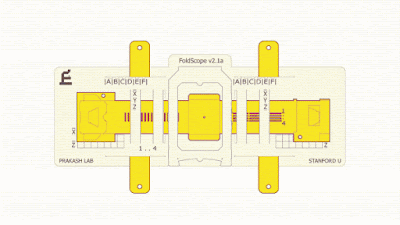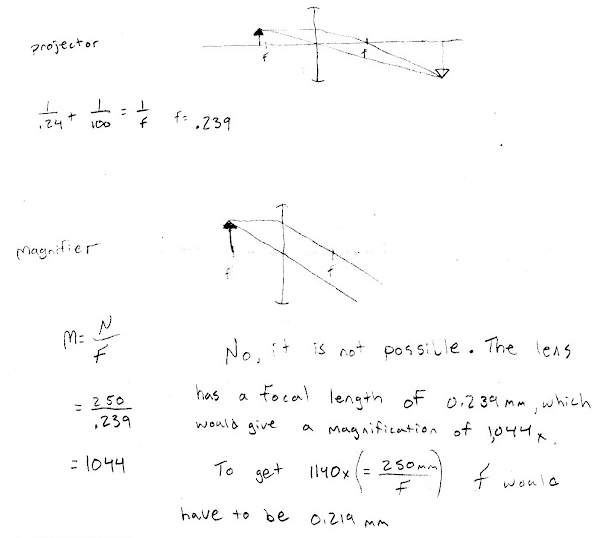## 20140329

### Physics midterm problem: Foldscope magnification claim

Physics 205B Midterm 1, spring semester 2014
Cuesta College, San Luis Obispo, CA

Cf. Giambattista/Richardson/Richardson, Physics, 2/e, Problems 23.57, 24.29"Manu Prakash: A 50-cent microscope that folds like origami"
youtu.be/h8cF5QPPmWU
TED Talks

The Foldscope is a low-cost "origami-based paper microscope"[*] designed by Stanford University researchers to be used for science, medicine, and education in developing countries.[**] It has a single converging lens that can either project an enlarged real image on a screen, or be used as a simple magnifier. When used as a projector, the object distance and image distances are 0.24 mm and 100.00 mm, respectively. When used as a simple magnifier, determine whether or not it is plausible that the angular magnification obtained with this Foldscope could be as high as the stated value of 1,140×. (Use the nominal value of 250 mm for the near point.) Show your work and explain your reasoning.

[*] "MAG (angular magnification) = 1,140×," arxiv.org/pdf/1403.1211v1.pdf.
[**] ted.com/talks/manu_prakash_a_50_cent_microscope_that_folds_like_origami.

• p:
Correct. Determines (a) the focal length of the lens using the thin lens equation, and (b) the resulting angular magnification when used as a simple magnifier, which is slightly less (1,044×) than the stated value (1,140×). May either argue that claim is plausible or implausible with these values.
• r:
Nearly correct, but includes minor math errors.
• t:
Nearly correct, but approach has conceptual errors, and/or major/compounded math errors. At least has focal length of lens, but compares linear magnification of projected real image instead of angular magnification of virtual image when used as a simple magnifier.
• v:
Implementation of right ideas, but in an inconsistent, incomplete, or unorganized manner.
• x:
Implementation of ideas, but credit given for effort rather than merit. Use of other optics equations, etc.
• y:
Irrelevant discussion/effectively blank.
• z:
Blank.
Sections 30882, 30883
Exam code: midterm01b0w7
p: 29 students
r: 2 students
t: 4 students
v: 6 students
x: 0 students
y: 0 students
z: 0 students

A sample "p" response (from student 7810):Another sample "p" response (from student 2419):